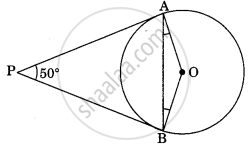# In the figure, if PA and PB are tangents to the circle with centre O such that ∠APB = 50°, then ∠AOB is equal to ______ - Mathematics

MCQ
Fill in the Blanks

In the figure, if PA and PB are tangents to the circle with centre O such that ∠APB = 50°, then ∠AOB is equal to ______• 25°

• 30°

• 40°

• 50°

#### Solution

In the figure, if PA and PB are tangents to the circle with centre O such that ∠APB = 50°, then ∠AOB is equal to 25°.

Explanation:

Given: A circle with center O and PA and PB are tangents to circle from a common external point P to point A and B respectively and ∠APB = 50°

To find: ∠OAB

OA ⏊ AP and OB ⏊ PB  .......[As tangent to at any point on the circle is perpendicular to the radius through point of contact]

∠OBP = ∠OAP = 90°   ......

∠OBP + ∠OAP + ∠AOB + ∠APB = 360°

90° + 90° + ∠AOB + 50° = 360°

∠AOB = 130°  .......

Now in ΔOAB

OA = OB   .......[Radii of same circle]

∠OBA = ∠OAB  .......

Also, By angle sum property of triangle

∠OBA + ∠OAB + ∠AOB = 180°

∠OAB + ∠OAB + 130° = 180°   ......[Using 2 and 3]

2∠OAB = 50°

∠OAB = 25°

Concept: Tangent to a Circle
Is there an error in this question or solution?

#### APPEARS IN

NCERT Mathematics Exemplar Class 10
Chapter 9 Circles
Exercise 9.1 | Q 8 | Page 103
Share